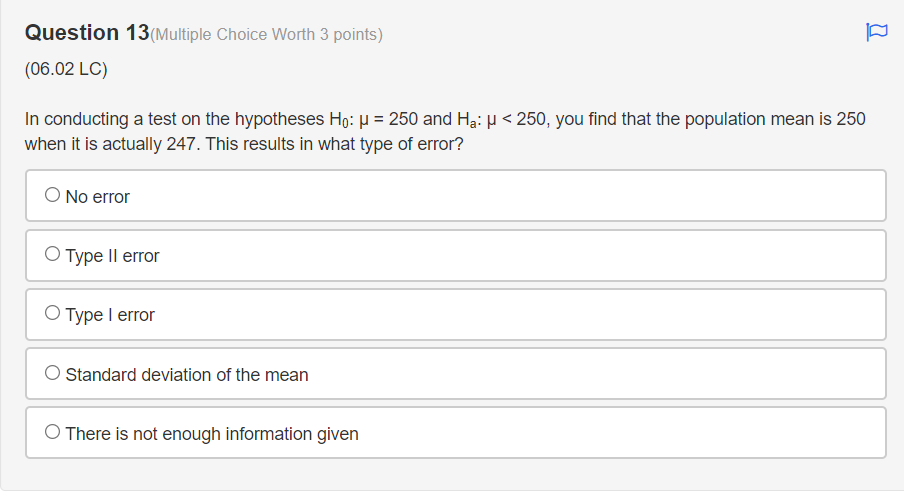# Question Question 13(Multiple Choice Worth 3 points) (06.02 LC) In conducting a test on the hypotheses Ho: = 250 and Ha: u < 250, you find that the population mean is 250 when it is actually 247. This results in what type of error? No error Type II error Type I error Standard deviation of the mean There is not enough information givenPZNZWH The Asker · Probability and StatisticsTranscribed Image Text: Question 13(Multiple Choice Worth 3 points) (06.02 LC) In conducting a test on the hypotheses Ho: = 250 and Ha: u < 250, you find that the population mean is 250 when it is actually 247. This results in what type of error? No error Type II error Type I error Standard deviation of the mean There is not enough information given
More
Transcribed Image Text: Question 13(Multiple Choice Worth 3 points) (06.02 LC) In conducting a test on the hypotheses Ho: = 250 and Ha: u < 250, you find that the population mean is 250 when it is actually 247. This results in what type of error? No error Type II error Type I error Standard deviation of the mean There is not enough information given